oxon88

## Homework Statement

The simply supported beam shown is 10 m long with
E = 200 × 109 Pa and I = 150 × 10–6 m4.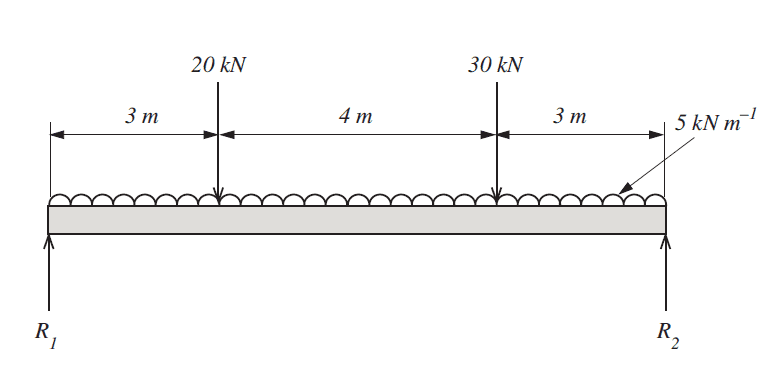1. Determine the position and magnitude of the maximum bending
moment.

2. Plot a graph of the deflection along the length of the beam
(calculate the deflection at 1 m intervals).

3. What I value for the beam would be required to halve the maximum
deflection of the beam?

4. The calculation for the maximum bending moment is to be verified
experimentally using a strain gauge bonded to the outer surface of the
beam, at the point where the maximum bending moment occurs.
Derive an equation which could be used to calculate the bending
moment from the measured strain value. State the meaning of all

## Homework Equations

M/I = σ/y = E/R

d2y / dx2 = M/EI

can anyone advise on where i should start?

Thanks.

Staff Emeritus
Homework Helper
1. Set up a free body diagram for the beam.
2. Solve for the unknown support reactions.
3. Draw the shear diagram for the beam.
4. Use the shear diagram to construct the bending moment diagram.
5. Once the moment diagram is done, make the M/I diagram.
6. Integrate the M/I diagram twice, making sure you keep the constants of integration.
7. After doing the integrations, apply the boundary conditions for the beam to determine the constants of integration.
8. Adjust the last integration to calculate the deflections of the beam.

oxon88

Is the free body diagram not the same as the attached diagram?

Staff Emeritus
Homework Helper
Yes.

oxon88
Ok I think i have calculated the unknown support reactions. does this look correct?

(3 * 20) + (7 * 30) + (5 * 10 *5) = r2 * 10

520 = r2 * 10

r2 = 52kN

upward forces=downward forces

r1 + 52 = 20 + 30 + (5 * 10)

r1 = 48kN

Staff Emeritus
Homework Helper
Looks good so far.

pongo38
oxon88, you didn't have to ask us if it was correct. You could check it yourself by taking moments about any axis other than the one you have already used going through R1.

oxon88
Thanks. I have drawn the shear force diagram and also calculated the bending moments (below).

x=0 = 0kN-m
x=1 = (48*1)-(5*1*0.5) = 45.5kN-m
x=2 = (48*2)-(5*2*1) = 86kN-m
x=3 = (48*3)-(5*3*1.5) = 121.5kN-m
x=4 = (48*4)-(20*1)-(5*4*2) = 132kN-m
x=5 = (48*5)-(20*2)-(5*5*2.5) = 137.5kN-m
x=6 = (48*6)-(20*3)-(5*6*3) = 138kN-m
x=7 = (48*7)-(20*4)-(5*7*3.5) = 133.5kN-m
x=8 = (48*8)-(20*5)-(30*1)-(5*8*4) = 94kN-m
x=9 = (48*9)-(20*6)-(30*2)-(5*9*4.5) = 49.5kN-m
x=10 = (48*10)-(20*7)-(30*3)-(5*10*5) = 0kN-m

Last edited:
Staff Emeritus
Homework Helper
I'm not sure what you are doing in Post #8.

At x = 0, the shear force diagram has a value equal to R1, or 48 kN. The bending moment at x = 0 is equal to 0 kN-m (Note the units).

Can you clarify?

oxon88
post #8 are my calculations for the bending moments along the beam. I have amended the units.

Staff Emeritus
Homework Helper
The bending moments look good. Proceed with answering the questions in the OP.

oxon88
thanks. Please can you explain what the M/I diagram is?

Last edited:
Staff Emeritus
Homework Helper
Just what it says. Take the ordinates of your bending moment diagram and divide by the moment of inertia of the beam (in this case I = 150 * 10^-6 m^4 according to the OP). It's not really required for this problem, since I is a constant, but when I changes along the length of the beam, an M/I diagram is useful when calculating deflection.

oxon88
x=0 = 0kN-m
x=1 = 45.5kN-m / 150 * 10^-6 m^4 = 303333.3
x=2 = 86kN-m / 150 * 10^-6 m^4 = 573333.3
x=3 = 121.5kN-m / 150 * 10^-6 m^4 = 810000
x=4 = 132kN-m / 150 * 10^-6 m^4 = 880000
x=5 = 137.5kN-m / 150 * 10^-6 m^4 = 916666.7
x=6 = 138kN-m / 150 * 10^-6 m^4 = 920000
x=7 = 133.5kN-m / 150 * 10^-6 m^4 = 890000
x=8 = 94kN-m / 150 * 10^-6 m^4 = 626666.7
x=9 = 49.5kN-m / 150 * 10^-6 m^4 = 330000
x=10 = 0kN-m

so would the diagram be as pictured?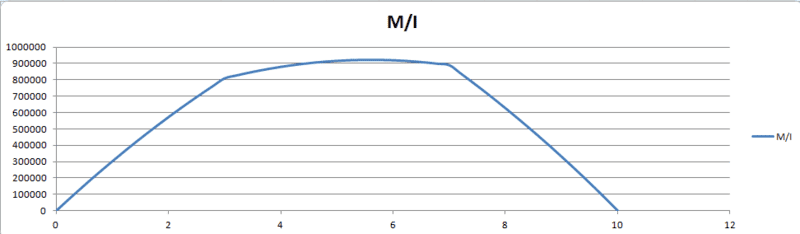Staff Emeritus
Homework Helper
Looks OK. Make sure you use the proper units.

•1 person
oxon88
ok Thankyou. What units should be used? Can you provide some guidance on how to integrate the M/I diagram twice?

Staff Emeritus
Homework Helper
ok Thankyou. What units should be used? Can you provide some guidance on how to integrate the M/I diagram twice?

Obviously, the units which result from dividing bending moment M by moment of inertia I.

The integration of the M/I diagram proceeds just like any other numerical integration: divide the x-axis into regular spacings and determine the M/I ordinates. You can use a trapezoidal rule to calculate the actual values of the integrals.

Remember, you must include the constants of integration in your calculation. There will be two unknown constants which are determined by applying the support conditions for your beam. You can start the integration by assuming the value of the integrals is zero at x = 0.

Since I is constant, the slope $\vartheta$ = (1/EI) ∫ M dx + C1
The deflection δ = (1/EI)∫∫ M dx + C1*x + C2

The final values for slope and deflection are determined by applying these constants of integration to your calculations of the integral values at the various x-locations.

oxon88
am i not able to use this formula to solve y?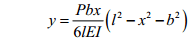Staff Emeritus
Homework Helper
am i not able to use this formula to solve y?

View attachment 63703

Where did you get this formula? It looks like only a point load P is present. What about deflection due to the distributed load?

oxon88
Our learning material gives it as an example. Yes it states that the formula is used for simply supported beams at ends with concentrated load P at any point.

Would it not be posssible to calculate the deflection for each of the loads and then sum the answers?

For the UDL, could this formula not be used?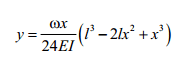Staff Emeritus
Homework Helper
Yes, you can use these formulas as long as you understand how to apply them properly.

oxon88
ok thanks. So i can work out the deflection for each of the loads, then sum them all together?

Do you think Macaulay’s Method would be a better method to use?

oxon88
I have got the following answers for total deflection. do these look feasible?

0m = 0mm
1m = 15.20mm
2m = 28.9mm
3m = 39.75mm
4m = 46.67mm
5m = 49.20mm
6m = 47.17mm
7m = 40.55mm
8m = 29.66mm
9m = 15.65mm
10m = 0mm

Last edited:
Staff Emeritus
Homework Helper
ok thanks. So i can work out the deflection for each of the loads, then sum them all together?

Yes. That's what superposition is all about w.r.t. beams.

Do you think Macaulay’s Method would be a better method to use?

It's up to you. You already have your deflection formulas. I don't think you would see any savings in terms of calculating the deflections as asked in the OP.

oxon88
one thing i cant understand....

when i work out δmax for the 20kN load. i get δmax=10.9033mm

however when working out the deflection in terms of x, i can see that at 4.1m δ = 11.051 mm

have i done something wrong?

δmax = =20000*7*((100-49)^1.5)/(9*(SQRT(3)*10*30000000))*1000 = 10.9033mm

δx4.1 = (20000*3*(4.1-10)/(6*10*30000000))*(9-2*10*4.1+4.1*4.1)*1000 = 11.0507mm

Staff Emeritus
Homework Helper
one thing i cant understand....

when i work out δmax for the 20kN load. i get δmax=10.9033mm

however when working out the deflection in terms of x, i can see that at 4.1m δ = 11.051 mm

have i done something wrong?

δmax = =20000*7*((100-49)^1.5)/(9*(SQRT(3)*10*30000000))*1000 = 10.9033mm

δx4.1 = (20000*3*(4.1-10)/(6*10*30000000))*(9-2*10*4.1+4.1*4.1)*1000 = 11.0507mm

It doesn't look like you have used the proper formula to calculate δx4.1

It doesn't match what you showed in an earlier post and it doesn't match the formula on this table:

pongo38
Note that because the beam is unsymetrically loaded, the maximum bending moment does not coincide with the geometric centre of the beam. The same is true for the maximum deflection. The point of maximum deflection is not necessarily the same as the point of maximum bending moment. If your formula is correct, you can presumably differentiate it to find the point of maximum deflection. It is worth pointing out that your calculations show a precision which no engineer would believe. This is because, in addition to deflection due to bending - given by the formula quoted-, there is also a further deflection (perhaps 2 or 3% more, depending on the material used) due to shear.

oxon88
It doesn't look like you have used the proper formula to calculate δx4.1

It doesn't match what you showed in an earlier post and it doesn't match the formula on this table:

im a little confused as to which formula to use. could you provide some guidance?

for x=4.1m,Should i be using this formula?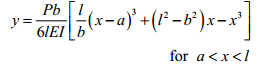or this one?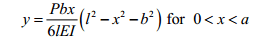Staff Emeritus
Homework Helper
For the 20 kN load, a = 3 m. You want to calculate the deflection at x = 4.1 m. This should make it easy to select the correct formula.

•1 person
oxon88
ok got it. here are my answers for the deflection.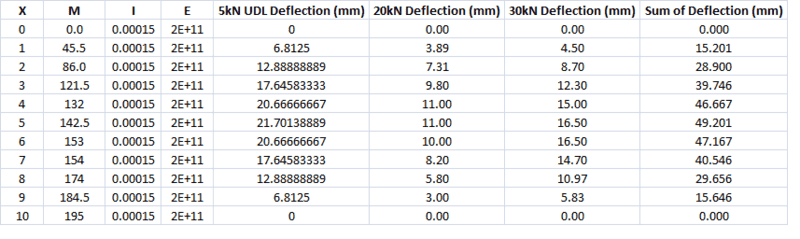and the graph looks like this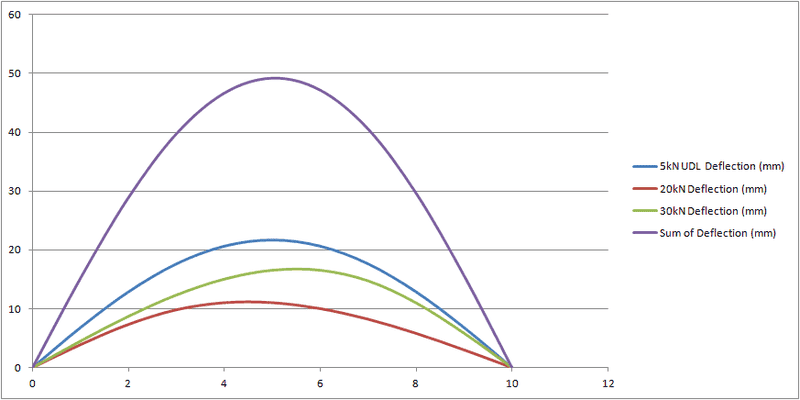does this look reasonable? Would the 5kN UDL really cause more of a deflection that the 30kN point load?

Last edited:
Staff Emeritus
Homework Helper
All you have to do is compare the expressions for deflection for a point load versus the distributed load.

•1 person
oxon88
ok Thank you. so my figures for deflection look acceptable?

Staff Emeritus
Homework Helper
Yes.

•1 person
oxon88
Q3. What I value for the beam would be required to halve the maximum
deflection of the beam?

A. In order to halve the maximum deflection of the beam, the value of I would need to be doubled to 300x10-6m4, this is because the deflection δ is inversely proportional to I

oxon88
does the above answer look acceptable?